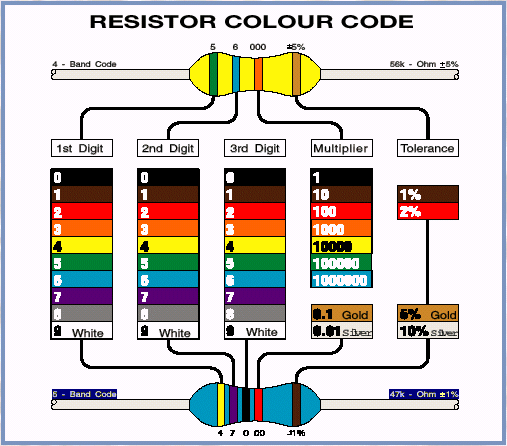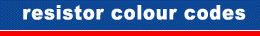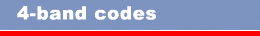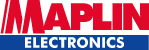Wirral & District Amateur Radio Club Resistor Colour CodeNote: On all of the colour-coded resistors, the band at one end will be spaced further apart than the others; the resistor should be viewed with this band to the right to correspond with the chart and examples.Reading from the left, bands 1 & 2 are the significant digits [1st green = 5, 2nd blue = 6]. Band 3 is the multiplier [orange = x1000]. Therefore the value of our example is 56 x 1000 ohms or 56k. The 4th band indicates the Tolerance [gold = ±5%].Reading from the left, bands 1, 2 & 3 are the significant digits [1st yellow = 4, 2nd violet = 7, 3rd black = 0]. Band 4 is the multiplier [red = x100]. Therefore the value of our example is 470 x 100 ohms or 47k. The 5th band indicates the Tolerance [brown = ±1%].
 Resistors resist the flow of electricity and are very useful in supplying desired voltages to other electronic components. The resistance of a resistor is measured in Ohms (). Please note that our Min Res resistors are usually supplied with the 4-Band Code, but with an additional red band to indicate the 50ppm temperature coefficient after the brown 1% tolerance band. This additional band prevents the code being read backwards accidentally, since no value begins red, brown (21—), in the event that the extra large space between the third and fourth bands is hard to identify. Most resistors are so small that it is impossible to print their values on them using normal numbers. Instead they are marked with a code of coloured bands. Resistors with a tolerance of 5% and 10% are marked with 4 bands, while higher precision types, such as 2%, 1% and better, may be marked with 5 bands to allow for an extra digit of precision.

Previous Page

Reproduced here by kind permission of